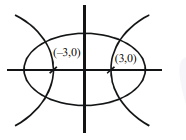# A hyperbola passes through the foci of the ellipse

Question:

A hyperbola passes through the foci of the ellipse $\frac{x^{2}}{25}+\frac{y^{2}}{16}=1$ and its transverse and conjugate axes coincide with major and minor axes of the ellipse, respectively. If the product of their eccentricities in one, then the equation of the hyperbola is :

1. $\frac{x^{2}}{9}-\frac{y^{2}}{25}=1$

2. $\frac{x^{2}}{9}-\frac{y^{2}}{16}=1$

3. $x^{2}-y^{2}=9$

4. $\frac{x^{2}}{9}-\frac{y^{2}}{4}=1$

Correct Option: , 2

Solution:For ellipse $e_{1}=\sqrt{1-\frac{b^{2}}{a^{2}}}=\frac{3}{5}$

for hyperbola $\mathrm{e}_{2}=\frac{5}{3}$

Let hyperbola be

$\frac{x^{2}}{a^{2}}-\frac{y^{2}}{b^{2}}=1$

$\because$ it passes through $(3,0) \Rightarrow \frac{9}{\mathrm{a}_{2}}=1$

$\Rightarrow \mathrm{a}^{2}=9$

$\Rightarrow \mathrm{b}^{2}=\mathrm{a}^{2}\left(\mathrm{e}^{2}-1\right)$

$=9\left(\frac{25}{9}-1\right)=16$

$\therefore$ Hyperbola is

$\frac{x^{2}}{9}-\frac{y^{2}}{16}=1$ ... option 2 .